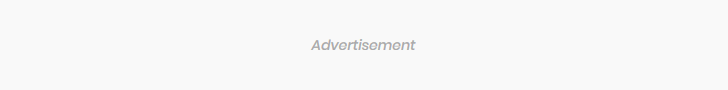### Subscribe Us# Indices (Surd) (CIE)

$\def\D{\displaystyle}$

1 (CIE 2012, s, paper 12, question 6)
You must not use a calculator in this question.
(i) Express $\D \frac{8}{\sqrt{3}+1}$ in the form $\D a(\sqrt{3}-1),$ where $\D a$ is an integer. 
An equilateral triangle has sides of length $\D \frac{8}{\sqrt{3}+1}.$
(ii) Show that the height of the triangle is $\D 6 - 2\sqrt{3} .$ 
(iii) Hence, or otherwise, find the area of the triangle in the form $\D p\sqrt{3} - q,$ where $\D p$ and $\D q$ are integers. 

2 (CIE 2012, s, paper 21, question 2)
A cuboid has a square base of side $\D (2 + \sqrt{3} )$ cm and a volume of $\D (16 + 9\sqrt{3} )$ cm$\D ^3.$ Without using a calculator, find the height of the cuboid in the form $\D (a + b\sqrt{3} )$ cm, where $\D a$ and $\D b$ are integers. 

3 (CIE 2012, w, paper 11, question 7)
Do not use a calculator in any part of this question.
(a) (i) Show that $\D 3\sqrt{5} - 2 \sqrt{2}$ is a square root of $\D 53 - 12\sqrt{10}.$ 
(ii) State the other square root of $\D 53 - 12\sqrt{10}.$ 
(b) Express $\D \frac{6\sqrt{3}+7\sqrt{2}}{4\sqrt{3}+5\sqrt{2}}$ in the form $\D a + b \sqrt{6},$ where $\D a$ and $\D b$ are integers to be found. 

4 (CIE 2012, w, paper 12, question 6)
Using $\D \sin15^{\circ} =\frac{\sqrt{2}}{4}(\sqrt{3}-1)$  and without using a calculator, find the value of $\D \sin\theta$ in the form $\D a + b \sqrt{2},$ where $\D a$ and $\D b$ are integers. 

5 (CIE 2012, w, paper 23, question 3)
Without using a calculator, simplify $\D \frac{(3\sqrt{3}-1)^2}{2\sqrt{3}-3},$  giving your answer in the form $\D \frac{a\sqrt{3}+b}{3},$ where $\D a$ and $\D b$ are integers. 

6 (CIE 2013, s, paper 11, question 7)
Calculators must not be used in this question.
The diagram shows a triangle $\D ABC$ in which angle $\D A = 90^{\circ}.$ Sides $\D AB$ and $\D AC$ are $\D \sqrt{5} - 2$ and $\D \sqrt{5} + 1$ respectively. Find
(i) $\D \tan B$ in the form $\D a + b\sqrt{5},$ where $\D a$ and $\D b$ are integers, 
(ii) $\D \sec^2B$ in the form $\D c + d \sqrt{5},$ where $\D c$ and $\D d$ are integers. 

7 (CIE 2013, s, paper 22, question 5) Fig
The diagram shows a trapezium $\D ABCD$ in which $\D AD = 7$ cm and $\D AB =(4+\sqrt{5})$ cm. $\D AX$ is perpendicular to $\D DC$ with $\D DX = 2$ cm and $\D XC = x$ cm. Given that the area of trapezium $\D ABCD$ is $\D 15(\sqrt{5}+2)$ cm$\D ^2,$ obtain an expression for $\D x$ in the form $\D a + b \sqrt{5},$ where $\D a$ and $\D b$ are integers. 

8 (CIE 2013, w, paper 21, question 2)
Do not use a calculator in this question.
Express $\D \frac{(4\sqrt{5}-2)^2}{\sqrt{5}-1}$  in the form $\D p \sqrt{5} + q,$ where $\D p$ and $\D q$ are integers. 

9 (CIE 2014, s, paper 21, question 2)
Without using a calculator, express $\D 6(1+\sqrt{3})^{-2}$  in the form $\D a + b \sqrt{3},$ where $\D a$ and $\D b$ are integers to be found. 

10 (CIE 2014, s, paper 22, question 1)
Without using a calculator, express $\D \frac{(2+\sqrt{5})^2}{\sqrt{5}-1}$  in the form $\D a + b\sqrt{5},$ where $\D a$ and $\D b$ are constants to be found. 

11 (CIE 2014, s, paper 23, question 5)
Do not use a calculator in this question.
(i) Show that $\D (2\sqrt{2}+4)^2-8(2\sqrt{2}+3)=0.$ 
(ii) Solve the equation $\D (2\sqrt{2}+3)x^2-(2\sqrt{2}+4)x+2=0,$ giving your answer in the form $\D a + b\sqrt{2}$ where $\D a$ and $\D b$ are integers. 

12 (CIE 2014, w, paper 21, question 9)
Integers $\D a$ and $\D b$ are such that $\D (a+ 3\sqrt{5} )^2+ a- b\sqrt{5}= 51.$  Find the possible values of $\D a$ and the corresponding values of $\D b.$ 

1.(i) $\D a=4$
(ii)
(iii) $\D 16\sqrt{3}-24$
2. $\D 4-\sqrt{3}$
3.(a)(i)
(ii) $\D -3\sqrt{5}+2\sqrt{2}$
(b) $\D -1+\sqrt{6}$
4. $\D 6-4\sqrt{2}$
5. $\D \frac{38\sqrt{3}}{3}$
6.(i) $\D 7+3\sqrt{5}$
(ii) $\D 95+42\sqrt{5}$
7. $\D 4+3\sqrt{5}$
8. $\D 17\sqrt{5}+1$
9. $\D 6-3\sqrt{3}$
10. $\D \frac{29}{4}+\frac{13}{4}\sqrt{5}$
11. $\D 2-\sqrt{2}$
12. $\D a=-3,2:b=-18,12$